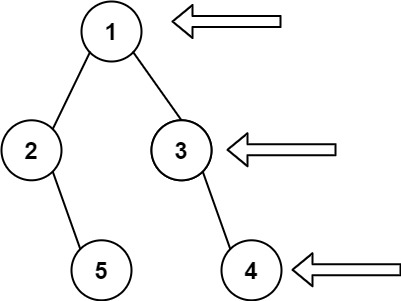# GeetCode Hub

Given the `root` of a binary tree, imagine yourself standing on the right side of it, return the values of the nodes you can see ordered from top to bottom.

Example 1:```Input: root = [1,2,3,null,5,null,4]
Output: [1,3,4]
```

Example 2:

```Input: root = [1,null,3]
Output: [1,3]
```

Example 3:

```Input: root = []
Output: []
```

Constraints:

• The number of nodes in the tree is in the range `[0, 100]`.
• `-100 <= Node.val <= 100`

/** * Definition for a binary tree node. * public class TreeNode { * int val; * TreeNode left; * TreeNode right; * TreeNode() {} * TreeNode(int val) { this.val = val; } * TreeNode(int val, TreeNode left, TreeNode right) { * this.val = val; * this.left = left; * this.right = right; * } * } */ class Solution { public List<Integer> rightSideView(TreeNode root) { } }